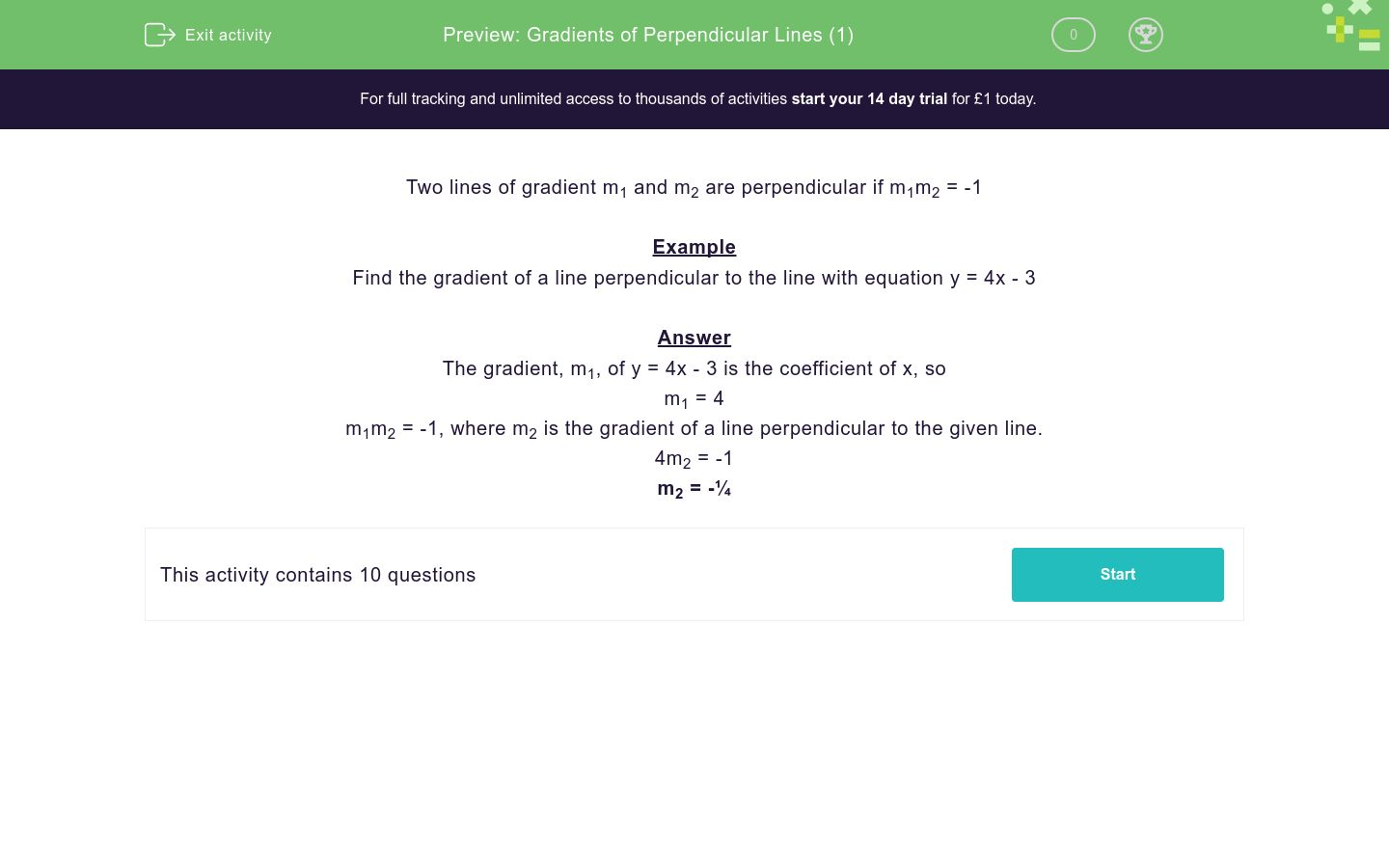# Gradients of Perpendicular Lines (1)

In this worksheet, students state the gradient of a line perpendicular to the given line.Key stage:  KS 4

Curriculum topic:  Algebra

Curriculum subtopic:  Use y = mx + c to Identify Parallel and Perpendicular Lines

Difficulty level:### QUESTION 1 of 10

Two lines of gradient m1 and m2 are perpendicular if m1m2 = -1

Example

Find the gradient of a line perpendicular to the line with equation y = 4x - 3

The gradient, m1, of y = 4x - 3 is the coefficient of x, so

m1 = 4

m1m2 = -1, where m2 is the gradient of a line perpendicular to the given line.

4m2 = -1

m2 = -¼

Find the gradient of a line perpendicular to the line with equation:

y = 3x - 4

1/3

-3

-1/3

Find the gradient of a line perpendicular to the line with equation:

y = -3x - 4

1/3

-3

-1/3

Find the gradient of a line perpendicular to the line with equation:

y = 4 - 3x

1/3

-1/4

-1/3

Find the gradient of a line perpendicular to the line with equation:

y = 2x + 4

1/2

-2

-1/2

Find the gradient of a line perpendicular to the line with equation:

y = -2x + 4

1/2

-2

-1/2

Find the gradient of a line perpendicular to the line with equation:

y = 7 - x

1

-1

0

Find the gradient of a line perpendicular to the line with equation:

y = 44 - 4x

4

1/4

-4

Find the gradient of a line perpendicular to the line with equation:

y = 44 + x

1

-1/44

-1

Find the gradient of a line perpendicular to the line with equation:

y = -5x

1/5

-1/5

5

Find the gradient of a line perpendicular to the line with equation:

x = 5

1/5

-1/5

0

• Question 1

Find the gradient of a line perpendicular to the line with equation:

y = 3x - 4

-1/3
EDDIE SAYS
3m2 = -1
• Question 2

Find the gradient of a line perpendicular to the line with equation:

y = -3x - 4

1/3
EDDIE SAYS
-3m2 = -1
• Question 3

Find the gradient of a line perpendicular to the line with equation:

y = 4 - 3x

1/3
EDDIE SAYS
-3m2 = -1
• Question 4

Find the gradient of a line perpendicular to the line with equation:

y = 2x + 4

-1/2
EDDIE SAYS
2m2 = -1
• Question 5

Find the gradient of a line perpendicular to the line with equation:

y = -2x + 4

1/2
EDDIE SAYS
-2m2 = -1
• Question 6

Find the gradient of a line perpendicular to the line with equation:

y = 7 - x

1
EDDIE SAYS
-m2 = -1
• Question 7

Find the gradient of a line perpendicular to the line with equation:

y = 44 - 4x

1/4
EDDIE SAYS
-4m2 = -1
• Question 8

Find the gradient of a line perpendicular to the line with equation:

y = 44 + x

-1
EDDIE SAYS
1m2 = -1
• Question 9

Find the gradient of a line perpendicular to the line with equation:

y = -5x

1/5
EDDIE SAYS
-5m2 = -1
• Question 10

Find the gradient of a line perpendicular to the line with equation:

x = 5

0
EDDIE SAYS
x = 5 is parallel to the y-axis
A line parallel to the x-axis has a gradient of 0
---- OR ----

Sign up for a £1 trial so you can track and measure your child's progress on this activity.

### What is EdPlace?

We're your National Curriculum aligned online education content provider helping each child succeed in English, maths and science from year 1 to GCSE. With an EdPlace account you’ll be able to track and measure progress, helping each child achieve their best. We build confidence and attainment by personalising each child’s learning at a level that suits them.